# Lua

## 简介

Lua 是一种轻量小巧的脚本语言，用标准C语言编写并以源代码形式开放， 其设计目的是为了嵌入应用程序中，从而为应用程序提供灵活的扩展和定制功能。

Lua 是巴西里约热内卢天主教大学（Pontifical Catholic University of Rio de Janeiro）里的一个研究小组于 1993 年开发的，该小组成员有：Roberto Ierusalimschy、Waldemar Celes 和 Luiz Henrique de Figueiredo。

## Lua 特性

1. 轻量级: 它用标准C语言编写并以源代码形式开放，编译后仅仅一百余K，可以很方便的嵌入别的程序里。
2. 可扩展: Lua提供了非常易于使用的扩展接口和机制：由宿主语言(通常是C或C++)提供这些功能，Lua可以使用它们，就像是本来就内置的功能一样。
3. 其它特性:
• 支持面向过程(procedure-oriented)编程和函数式编程(functional programming)；
• 自动内存管理；只提供了一种通用类型的表（table），用它可以实现数组，哈希表，集合，对象；
• 语言内置模式匹配；闭包(closure)；函数也可以看做一个值；提供多线程（协同进程，并非操作系统所支持的线程）支持；
• 通过闭包和table可以很方便地支持面向对象编程所需要的一些关键机制，比如数据抽象，虚函数，继承和重载等。

## Lua 应用场景

• 游戏开发
• 独立应用脚本
• Web 应用脚本
• 扩展和数据库插件如：MySQL Proxy 和 MySQL WorkBench
• 安全系统，如入侵检测系统

GitHub

## Lua和C#的区别

Lua可以在几乎所有的操作系统和平台进行编译运行

C#只能在特定的操作系统中进行编译成dll文件，然后打包进安装包在其他平台（Android、iOS）运行

# 数据类型

Lua 是动态类型语言，变量不要类型定义,只需要为变量赋值。 值可以存储在变量中，作为参数传递或结果返回。

Lua 中有 8 个基本类型分别为：nil、boolean、number、string、userdata、function、thread 和 table。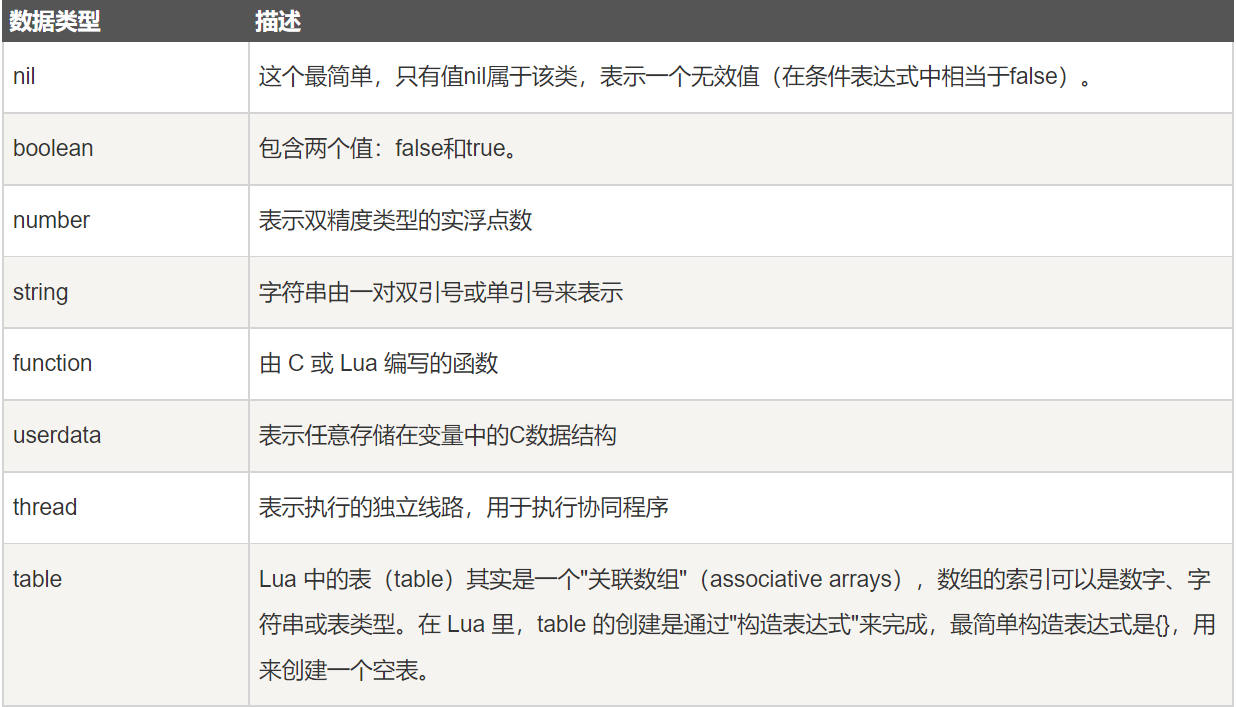print(type("Hello world"))      --> string
print(type(10.4*3))             --> number
print(type(print))              --> function
print(type(type))               --> function
print(type(true))               --> boolean
print(type(nil))                --> nil
print(type(type(X)))            --> string


## nil

nil 类型表示一种没有任何有效值，它只有一个值 – nil，例如打印一个没有赋值的变量，便会输出一个 nil 值：

> print(type(a))
nil
>


tab1 = { key1 = "val1", key2 = "val2", "val3" }
for k, v in pairs(tab1) do
print(k .. " - " .. v)
end

print()

tab1.key1 = nil
for k, v in pairs(tab1) do
print(k .. " - " .. v)
end

console:
1 - val3
key1 - val1
key2 - val2

1 - val3
key2 - val2


nil 作比较时应该加上双引号 "：

> type(X)
nil
> type(X)==nil
false
> type(X)=="nil"
true
>


type(x) == nil 结果为 false 的原因是 type(x) 实质是返回的 “nil” 字符串，是一个 string 类型：

print(type(x))
print(type(x) == nil)
print(type(x) == "nil")
print(type(type(x)))

console:
nil
false
true
string


## boolean

boolean 类型只有两个可选值：true（真） 和 false（假），Lua 把 false 和 nil 看作是 false，其他的都为 true，数字 0 也是 true。

print(type(true))
print(type(false))
print(type(nil))

if false or nil then
print("至少有一个是 true")
else
print("false 和 nil 都为 false")
end

if 0 then
print("数字 0 是 true")
else
print("数字 0 为 false")
end

console:
boolean
boolean
nil
false 和 nil 都为 false



## number

Lua 默认只有一种 number 类型 – double（双精度）类型（默认类型可以修改 luaconf.h 里的定义），以下几种写法都被看作是 number 类型：

print(type(2))
print(type(2.2))
print(type(0.2))
print(type(2e+1))
print(type(0.2e-1))
print(type(7.8263692594256e-06))

console:
number
number
number
number
number
number


## string

string1 = "this is string1"
string2 = 'this is string2'


html = [[
<html>
<body>
<a href="http://www.runoob.com/">菜鸟教程</a>
</body>
</html>
]]
print(html)

console:
<html>
<body>
<a href="http://www.runoob.com/">菜鸟教程</a>
</body>
</html>


> print("2" + 6)
8.0
> print("2" + "6")
8.0
> print("2 + 6")
2 + 6
> print("-2e2" * "6")
-1200.0
> print("error" + 1)
stdin:1: attempt to perform arithmetic on a string value
stack traceback:
stdin:1: in main chunk
[C]: in ?
>


> print("a" .. 'b')
ab
> print(157 .. 428)
157428
>


> len = "www.runoob.com"
> print(#len)
14
> print(#"www.runoob.com")
14
>


## function

-- function_test.lua 脚本文件
function factorial1(n)
if n == 0 then
return 1
else
return n * factorial1(n - 1)
end
end
print(factorial1(5))
factorial2 = factorial1
print(factorial2(5))

console:
120
120


function 可以以 匿名函数（anonymous function） 的方式通过参数传递:

-- function_test2.lua 脚本文件
function testFun(tab,fun)
for k ,v in pairs(tab) do
print(fun(k,v));
end
end

tab={key1="val1",key2="val2"};
testFun(tab,
function(key,val)--匿名函数
return key.."="..val;
end
);

console:
key1 = val1
key2 = val2


## userdata

userdata 是一种用户自定义数据，用于表示一种由应用程序或 C/C++ 语言库所创建的类型，可以将任意 C/C++ 的任意数据类型的数据（通常是 struct 和 指针）存储到 Lua 变量中调用。

## table

-- 创建一个空的 table
local tbl1 = {}

-- 直接初始表
local tbl2 = {"apple", "pear", "orange", "grape"}


Lua 中的表（table）其实是一个"关联数组"（associative arrays），数组的索引可以是数字或者是字符串。

a = {}
a["key"] = "value"
key = 10
a[key] = 22
a[key] = a[key] + 11
for k, v in pairs(a) do
print(k .. " : " .. v)
end

console:
key : value
10 : 33


local tbl = {"apple", "pear", "orange", "grape"}
for key, val in pairs(tbl) do
print("Key", key)
end

console:
Key    1
Key    2
Key    3
Key    4


table 不会固定长度大小，有新数据添加时 table 长度会自动增长，没初始的 table 都是 nil。

a3 = {}
for i = 1, 10 do
a3[i] = i
end
a3["key"] = "val"
print(a3["key"])
print(a3["none"])

console:
val
nil


# 变量

## 基本

• Lua 变量有三种类型：全局变量、局部变量、表中的域。
• Lua 中的变量全是全局变量，哪怕是语句块或是函数里，除非用 local 显式声明为局部变量。
• 局部变量的作用域为从声明位置开始到所在语句块结束。
• 变量的默认值均为 nil。
a = 5               -- 全局变量
local b = 5         -- 局部变量

function joke()
c = 5           -- 全局变量
local d = 6     -- 局部变量
end

joke()
print(c,d)          --> 5 nil

do
local a = 6     -- 局部变量
b = 6           -- 对局部变量重新赋值
print(a,b);     --> 6 6
end

print(a,b)      --> 5 6

console:
5    nil
6    6
5    6


## 赋值语句

a = "hello" .. "world"
t.n = t.n + 1


Lua 可以对多个变量同时赋值，变量列表和值列表的各个元素用逗号分开，赋值语句右边的值会依次赋给左边的变量。

a, b = 10, 2*x       <-->       a=10; b=2*x


x, y = y, x                     -- swap 'x' for 'y'
a[i], a[j] = a[j], a[i]         -- swap 'a[i]' for 'a[j]'


a. 变量个数 > 值的个数             按变量个数补足nil
b. 变量个数 < 值的个数             多余的值会被忽略

a, b, c = 0, 1
print(a,b,c)             --> 0   1   nil

a, b = a+1, b+1, b+2     -- value of b+2 is ignored
print(a,b)               --> 1   2

a, b, c = 0
print(a,b,c)             --> 0   nil   nil


a, b, c = 0, 0, 0
print(a,b,c)             --> 0   0   0


a, b = f()


f()返回两个值，第一个赋给a，第二个赋给b。

1. 避免命名冲突。
2. 访问局部变量的速度比全局变量更快。

## 索引

t[i]
t.i                 -- 当索引为字符串类型时的一种简化写法
gettable_event(t,i) -- 采用索引访问本质上是一个类似这样的函数调用

> site = {}
> site["key"] = "www.runoob.com"
> print(site["key"])
www.runoob.com
> print(site.key)
www.runoob.com


## 全局变量和nil

> print(b)
nil
> b=10
> print(b)
10
>


b = nil
print(b)      --> nil


# 运算符

• 算术运算符
• 关系运算符
• 逻辑运算符
• 其他运算符

## 算术运算符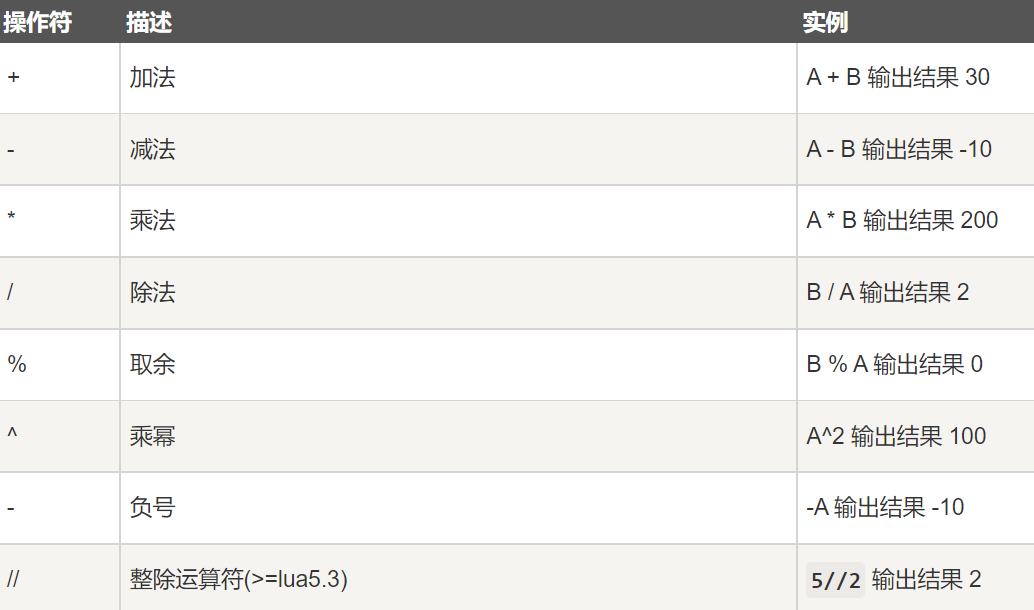a = 5
b = 2
print("除法运算 - a/b 的值为 ", a / b )
print("整除运算 - a//b 的值为 ", a // b )



## 关系运算符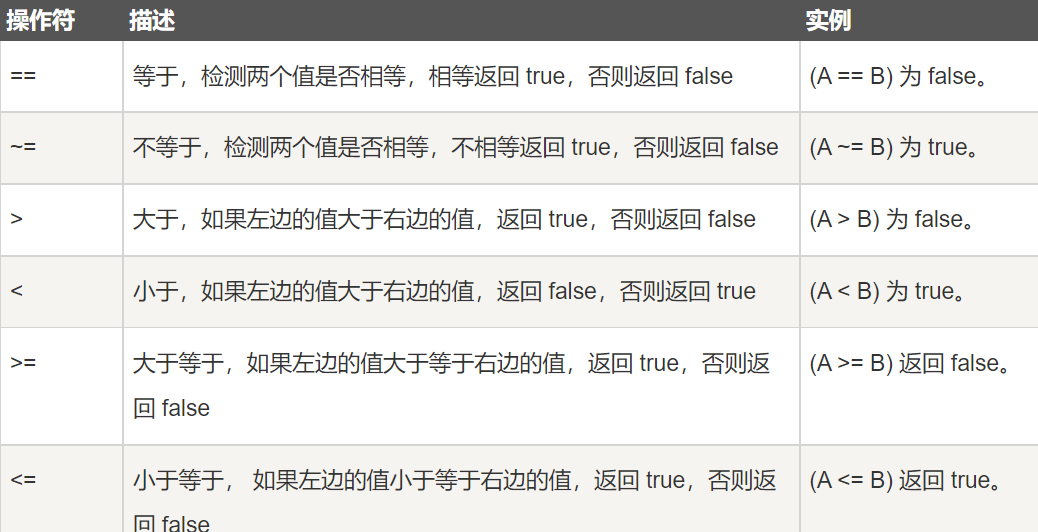## 逻辑运算符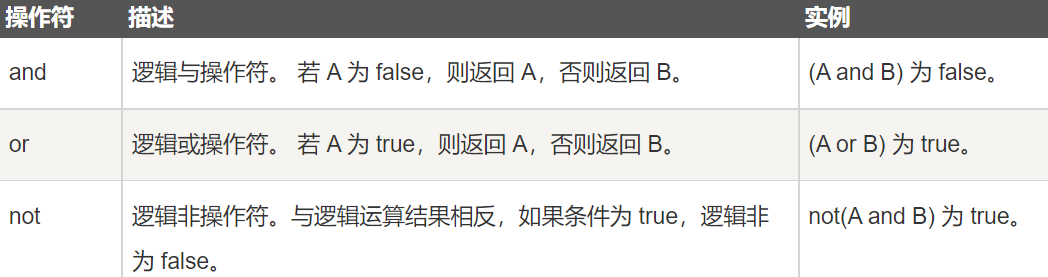## 其他运算符## 运算符优先级

^
not    - (unary)
*      /       %
+      -
..
<      >      <=     >=     ~=     ==
and
or


a+i < b/2+1          <-->       (a+i) < ((b/2)+1)
5+x^2*8              <-->       5+((x^2)*8)
a < y and y <= z     <-->       (a < y) and (y <= z)
-x^2                 <-->       -(x^2)
x^y^z                <-->       x^(y^z)


# 基本语法

## 注释

--


--[[
多行注释
多行注释
--]]


## 关键词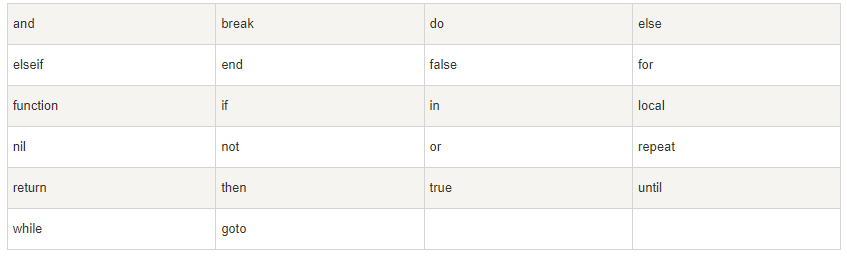## 循环

### while

while(condition)
do
statements
end


### for

Lua 编程语言中 for语句有两大类：

• 数值for循环
• 泛型for循环

#### 数值for循环

for var = exp1, exp2, exp3 do
<执行体>
end


var 从 exp1 变化到 exp2，每次变化以 exp3 为步长递增 var，并执行一次 “执行体”。exp3 是可选的，如果不指定，默认为1。

for i=1,f(x) do
print(i)
end

for i=10,1,-1 do
print(i)
end


for的三个表达式在循环开始前一次性求值，以后不再进行求值。 比如上面的f(x)只会在循环开始前执行一次，其结果用在后面的循环中。

function f(x)
print("function")
return x*2
end
for i=1,f(5) do print(i)
end

console:
function
1
2
3
4
5
6
7
8
9
10


#### 泛型for循环

Lua 编程语言中泛型 for 循环语法格式:

--打印数组a的所有值
a = {"one", "two", "three"}
for i, v in ipairs(a) do
print(i, v)
end

console:
1	one
2	two
3	three

days = {"Sunday","Monday","Tuesday","Wednesday","Thursday","Friday","Saturday"}
for i,v in ipairs(days) do
print(v)
end

console:
Sunday
Monday
Tuesday
Wednesday
Thursday
Friday
Saturday


### repeat…until

Lua 编程语言中 repeat…until 循环语句不同于 for 和 while循环，for 和 while 循环的条件语句在当前循环执行开始时判断，而 repeat…until 循环的条件语句在当前循环结束后判断。

repeat
statements
until( condition )


Lua repeat…until 循环流程图如下：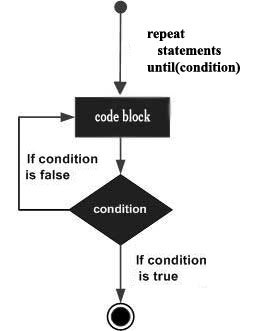--[ 变量定义 --]
a = 10
--[ 执行循环 --]
repeat
print("a的值为:", a)
a = a + 1
until( a > 15 )

a的值为:    10
a的值为:    11
a的值为:    12
a的值为:    13
a的值为:    14
a的值为:    15


## 循环控制语句

break 语句：退出当前循环或语句，并开始脚本执行紧接着的语句。
goto 语句：将程序的控制点转移到一个标签处。

### break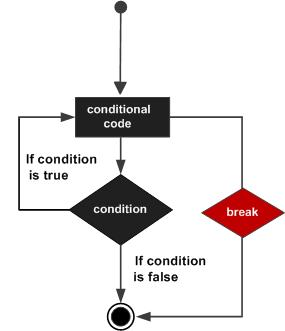### goto

local a = 1
::label:: print("--- goto label ---")

a = a+1
if a < 3 then
goto label   -- a 小于 3 的时候跳转到标签 label
end

--- goto label ---
--- goto label ---


for i=1, 3 do
if i <= 2 then
print(i, "yes continue")
goto continue
end
print(i, " no continue")
::continue::
print([[i'm end]])
end

1   yes continue
i'm end
2   yes continue
i'm end
3    no continue
i'm end


## 流程控制

if(布尔表达式)
then
--[ 在布尔表达式为 true 时执行的语句 --]
end

if(布尔表达式)
then
--[ 布尔表达式为 true 时执行该语句块 --]
else
--[ 布尔表达式为 false 时执行该语句块 --]
end

if( 布尔表达式 1)
then
--[ 在布尔表达式 1 为 true 时执行该语句块 --]

elseif( 布尔表达式 2)
then
--[ 在布尔表达式 2 为 true 时执行该语句块 --]

elseif( 布尔表达式 3)
then
--[ 在布尔表达式 3 为 true 时执行该语句块 --]
else
--[ 如果以上布尔表达式都不为 true 则执行该语句块 --]
end


# 函数

optional_function_scope function function_name( argument1, argument2, argument3..., argumentn)
function_body
return result_params_comma_separated
end


• optional_function_scope: 该参数是可选的制定函数是全局函数还是局部函数，未设置该参数默认为全局函数，如果你需要设置函数为局部函数需要使用关键字 local。
• function_name: 指定函数名称。
• argument1, argument2, argument3…, argumentn: 函数参数，多个参数以逗号隔开，函数也可以不带参数。
• function_body: 函数体，函数中需要执行的代码语句块。
• result_params_comma_separated: 函数返回值，Lua语言函数可以返回多个值，每个值以逗号隔开。

## 一个返回值

--[[ 函数返回两个值的最大值 --]]
function max(num1, num2)

if (num1 > num2) then
result = num1;
else
result = num2;
end

return result;
end
-- 调用函数
print("两值比较最大值为 ",max(10,4))
print("两值比较最大值为 ",max(5,6))



## 多个返回值

Lua函数可以返回多个结果值，比如string.find，其返回匹配串"开始和结束的下标"（如果不存在匹配串返回nil）。

function maximum (a)
local mi = 1             -- 最大值索引
local m = a[mi]          -- 最大值
for i,val in ipairs(a) do
if val > m then
mi = i
m = val
end
end
return m, mi
end

print(maximum({8,10,23,12,5}))

23    3


## 将函数作为形参

Lua 中我们可以将函数作为参数传递给函数，如下实例：

myprint = function(param)
print("这是打印函数 -   ##",param,"##")
end

result = num1 + num2
-- 调用传递的函数参数
functionPrint(result)
end
myprint(10)
-- myprint 函数作为参数传递

console:



## 可变参数

Lua 函数可以接受可变数目的参数，和 C 语言类似，在函数参数列表中使用三点 … 表示函数有可变的参数。

function add(...)
local s = 0
for i, v in ipairs{...} do   --> {...} 表示一个由所有变长参数构成的数组
s = s + v
end
return s
end


#变量名称 来获取一个代表表的变量中的元素个数

function average(...)
result = 0
local arg={...}    --> arg 为一个表，局部变量
for i,v in ipairs(arg) do
result = result + v
end
print("总共传入 " .. #arg .. " 个数")
return result/#arg
end

print("平均值为",average(10,5,3,4,5,6))



function average(...)
result = 0
local arg={...}
for i,v in ipairs(arg) do
result = result + v
end
print("总共传入 " .. select("#",...) .. " 个数")
return result/select("#",...)
end

print("平均值为",average(10,5,3,4,5,6))



function fwrite(fmt, ...)  ---> 固定的参数fmt
return io.write(string.format(fmt, ...))
end

fwrite("runoob\n")       --->fmt = "runoob", 没有变长参数。
fwrite("%d%d\n", 1, 2)   --->fmt = "%d%d", 变长参数为 1 和 2

runoob
12


select(‘#’, …) 返回可变参数的长度（数量）。
select(n, …) 用于返回从起点 n 开始到结束位置的所有参数列表。

function f(...)
a = select(3,...)  -->从第三个位置开始，变量 a 对应右边变量列表的第一个参数
print (a)
print (select(3,...)) -->打印所有列表参数
end

f(0,1,2,3,4,5)

2
2       3       4       5

do
function foo(...)
for i = 1, select('#', ...) do  -->获取参数总数
local arg = select(i, ...); -->读取参数，arg 对应的是右边变量列表的第一个参数
print("arg", arg);
end
end

foo(1, 2, 3, 4);
end

arg    1
arg    2
arg    3
arg    4

09-21
12-14
06-251126
04-13390
12-303650
04-222万+
05-131万+
10-053万+
08-216357
10-05
09-22
01-08
09-21
09-22

### “相关推荐”对你有帮助么？

•非常没帮助
•没帮助
•一般
•有帮助
•非常有帮助被折叠的  条评论 为什么被折叠?到【灌水乐园】发言YYYMarshal

¥2 ¥4 ¥6 ¥10 ¥20余额支付 (余额：-- )扫码支付获取中扫码支付点击重新获取扫码支付1.余额是钱包充值的虚拟货币，按照1:1的比例进行支付金额的抵扣。
2.余额无法直接购买下载，可以购买VIP、C币套餐、付费专栏及课程。余额充值